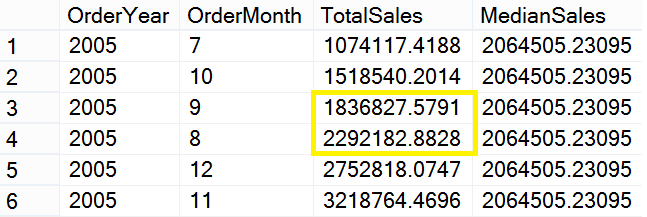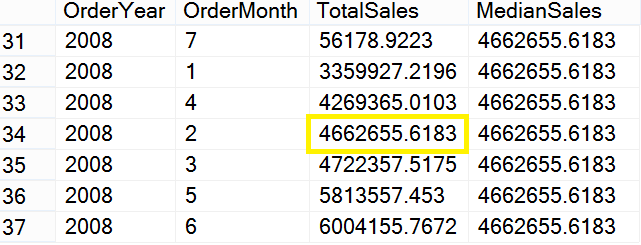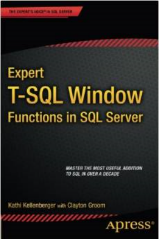# SQL SERVER – What are T-SQL Median? – Notes from the Field #090[Note from Pinal]: In this episode of the Notes from the Field series database expert Kathi Kellenberger explains about T-SQL Median. Kathi is an amazing instructor, she was the SQL author I have read in my early career. The reason, I love SQL Server because her writing has instigated love for this technology in me. Today she brings a relatively interesting topic for database experts. Read the experience of  Kathi in her own words.

SQL Server has a wealth of useful T-SQL functions that let you do just about anything you need to do. One function that is sorely missing is a MEDIAN function. A median is the middle value in a set of values. For example, 3 is the median of the set 1, 3, 5. If the set is even, the median is calculated or “interpolated” by averaging the two middle values. The median of this set 1, 3, 5, 7 is 4.

You will see median values used frequently even if you do not work with statistics yourself. For example, you could see a news story listing the median household income of a city by neighborhood, the median salary for developers by language, or the median age of a population.

As I mentioned, there is no MEDIAN function in T-SQL. If, however, you are fortunate enough to be running SQL Server 2012 or 2014, there is an easy workaround. In 2012, Microsoft introduced a new function called PERCENTILE_CONT, one of the T-SQL window functions. Given a percent rank and a set of values, PERCENTILE_CONT will return the value ranked at that percent. If there is not an exact value found at the rank, PERCENTILE_CONT will interpolate the answer instead. If you supply 0.5, meaning 50%, PERCENTILE_CONT will return the median value. Here is an example that calculates the median sales by month for each year:

`WITH Totals AS ( SELECT YEAR(OrderDate) AS OrderYear, MONTH(OrderDate) AS OrderMonth, SUM(TotalDue) AS TotalSales FROM Sales.SalesOrderHeader GROUP BY YEAR(OrderDate), MONTH(OrderDate) ) SELECT OrderYear, OrderMonth, TotalSales, PERCENTILE_CONT(0.5) WITHIN GROUP(ORDER BY TotalSales) OVER(PARTITION BY Totals.OrderYear) AS MedianSales FROM Totals ORDER BY OrderYear, TotalSales; `

Before we take a look at the results, let’s review the query. The query has a common table expression (CTE) that calculates the sales for each month and year. In the outer query, the PERCENTILE_CONT function is used with an argument of 0.5. PERCENTILE_CONT is a T-SQL window function, but the syntax for it and a similar function, PERCENTILE_DISC, is different than the other window functions. The function name is followed by the WITHIN GROUP clause. This is where the ORDER BY expression goes, not in the OVER clause. The ORDER BY expression is used by the function to line up the values so that the ranks can be compared to the percentile rank value provided as an argument. The OVER clause supports PARTITION BY, and in this case we would like to get the median within each year, so the PARTITION BY expression is OrderYear.

In 2005, there were orders placed between July and December, six months. Six is even, so the function calculates the average of two middle months as shown in Figure 1.Figure 1: The Median Sales for 2005

Every year in the data has an even number of months except for 2008. In 2008, there are seven months with sales, so the function can find an exact median value. Figure 2 shows the 2008 sales:Figure 2: The Median Sales for 2008

You may be wondering what the difference is between PERCENTILE_CONT and PERCENTILE_DISC. PERCENTILE_DISC always returns an exact value found in the set, never a calculation. For calculating the median, PERCENTILE_CONT is the function to use.If you would like to learn more about T-SQL window functions, be sure to check out my latest book Expert T-SQL Window Functions in SQL Server or my Pluralsight course T-SQL Window Functions.

If you want to get started with SQL Server with the help of experts, read more over at Fix Your SQL Server.

Reference: Pinal Dave (https://blog.sqlauthority.com)

#### Related Posts

•Joe Celko
July 23, 2015 7:42 am

Actually, there are several kinds of medians. I like to use the weighted media which I feel is a better measure of central tendency . Give the set {1,2,2,3,3,3} that statistical median is (2+3)/2 = 2.5. The weighted median uses all of the repeated values and (2+2+3+3+3) / 5 = 2.6; this show that the center is skewed to the right.

•Pinal Dave
July 24, 2015 6:05 am

As always, you are correct Joe.

•